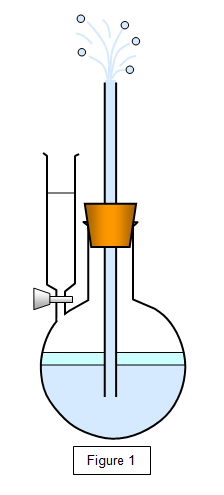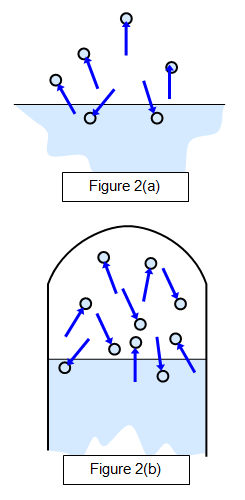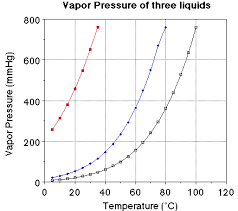Home PHYSICS TOPIC 8: VAPOUR AND HUMIDITY | PHYSICS FORM 3

# TOPIC 8: VAPOUR AND HUMIDITY | PHYSICS FORM 3

143
0
SHARE### TOPIC 8: VAPOUR AND HUMIDITY | PHYSICS FORM 3

Vapour

The Process of Evaporation of Liquid
Explain the process of evaporation of liquid
Vapours
• These are molecules which escape into the atmosphere after liquids are heated.
• When a liquid is heated strongly then molecules tends to escape ( those molecules are called vapour).
• Most liquids evaporates at any temperature however liquids may vary in the rate at which they evaporate at ordinary temperature.
• Alcohol and ether evaporate rapidly but lubricating oil and mercury hardly evaporate.
• Evaporation of a liquids result in the formation of vapour.
Factors Affecting Evaporation of a Liquid
Identify factors affecting evaporation of a liquid
There are several factors which affect evaporation of liquids when heated which include the following:
1. Nature of the liquid:
Normally liquids evaporation differs depending on the nature of liquid.
Example; Volatile liquids evaporate faster than non-volatile liquids,
which evaporate slowly. Alcohol evaporates faster than other liquids
like water. The boiling point of alcohol is 780C while that of water is 100oC.
2. Pressure above the liquid (atmospheric pressure): When the atmospheric pressure is high, the rate of evaporation may be reduced.
3. Surface energy of the liquid:
This forms a boundary or skin between the liquid and the atmosphere.
The surface energy prevents molecules with lower kinetic energy from
escaping into the atmosphere. Some liquids such as alcohol have low
surface energy , hence they evaporate rapidly.
Question Time 1
Why do molecules escapes when the liquid is heated?
When the liquid is heated, the molecules tend to gain (absorb) kinetic energy hence the random speed of the moleculesincreases.
The
process of evaporation of liquid can be explained using Kinetic
Theory.When a liquid is left to evaporate in a closed container, the
pressure of the vapour in the container gradually increases.
Difference between Saturated and Evaporation of a Liquid
Distinguish between saturated and evaporation of a liquid
Vapours
The
molecules in a liquid are in a state of continuous motion and some of
those at the liquid surface will gain sufficient energy to escape from
the surface altogether. The molecules that have left the surface are
said to be in the vapour state. The difference between a vapour and a
gas is purely one of temperature, a vapour being a gas below its
critical temperature.
This
phenomenon is known as evaporation. The number of molecules leaving the
surface, and hence the rate of evaporation, will increase with
temperature as the liquid contains more energy at a higher temperature.
The effect of the evaporation of a liquid can be shown clearly by the
following experiment.
Some
ether is run into the flask, as shown in the figure below. It will
evaporate in the enclosed space and the pressure that it exerts on the
water will force a jet of water out of the tube. Warming the liquid will
increase this evaporation and give a more powerful jet.You can show that the rate of evaporation may be increased by:
1. Warming the flask gently.
2. Increasing the area of the liquid surface.
3. Blowing a stream of air across the surface.
4. Reducing the pressure above the liquid surface.
Saturated vapours
When
a liquid is in a closed container the space above the liquid is full of
vapour, and the vapour is then described as a saturated vapour – this
means that the density of the liquid molecules in the air is a maximum.
This is due to molecules continually escaping and reentering the liquid.
At any moment the number of molecules leaving the surface will be equal
to the number returning to it and so a dynamic equilibrium is set up.
The
properties of saturated vapours were first investigated by Dalton
around 1800. This is shown in Figure 2(a), which shows a state before
saturation has been reached (when there will be more molecules leaving
the surface than returning to it) and Figure 2(b), which shows the
saturated state. A dynamic equilibrium exits here.This
vapour will exert a pressure and if there is sufficient liquid the air
above the liquid surface will be saturated with vapour; the pressure
that this saturated vapour exerts is known as the saturated vapour
pressure (s.v.p.) of the liquid at that temperature.
Notice
that since the velocity of the molecules increases with temperature the
saturated vapour pressure also increases with temperature, and
therefore the temperature of the vapour must be specified when quoting
its saturated vapour pressure (s.v.p.)
The Effect of Temperature on Saturated Vapour Pressure (S.V.P) of a Liquid
Explain the effect of temperature on saturated vapour pressure (S.V.P) of a liquid
Saturated vapour pressure (S.V.P):Is
the pressure exerted by vapour when a liquid is heated and reaches a
state of Equilibrium where eventually the rate at which the molecules
leave the liquid is equal to the rate at which others return to it.
The
Height, of mercury represents the saturated vapour pressure of the
liquid in the flask. Saturated vapour pressure increases with the
increase in Temperature (Ti) and the increased with decrease in
Temperature (Td).The graph of saturated vapour pressure (svp) against Temperature
The
graph shows as saturated vapour pressure (s.v.p) increases then the
temperature will increase and vice versa for decrease of temperature.
Boiling
point (B.P):Is the temperature reached where the saturated vapour
pressure (S.V.P) is equal to external atmospheric pressure.
The Boiling point of alcohol is 78OC water is 100OC
and pressure of the atmosphere as 76cm of mercury.The intersection of
the normal atmosphere pressure line with the liquids S.V.P curve.
SHARE